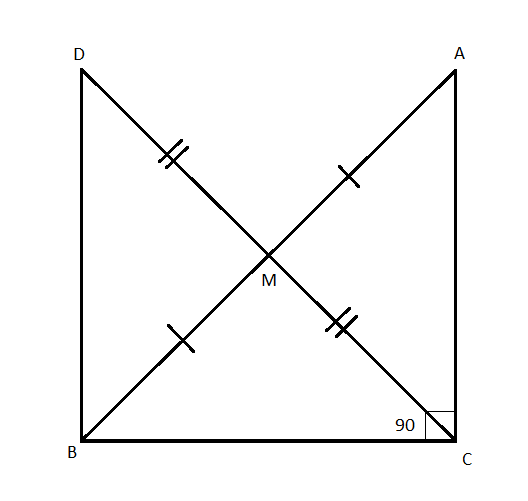QUESTION

# In a right triangle $ABC$, the right angle at $C,M$ is the midpoint of hypotenuse $AB$. $C$ is joined to $M$ and produced to a point $D$ such that $DM = CM$. Point $D$ is joined to point $B$.A. $\Delta AMC \cong \Delta BMD$B. $\Delta DBC$ is a right angleC. $\Delta DBC \cong \Delta ACB$D. $CM = \dfrac{1}{2}AB$

Hint: In this question first of all draw the figure by using the given data which will give us a clear idea of what we have to find. By using SAS congruence rules, the triangles AMC and BMD are similar triangles. So, use this concept to reach the solution of the given problem.

Given $\Delta ABC$ is a right-angled triangle at $C$.
So, $\angle ACB = {90^\circ}$
$M$ is the midpoint of $AB$
So, $AM = BM...................................\left( 1 \right)$
$DM = CM..........................................\left( 2 \right)$
By using the given data, the diagram will be as shown in the figure:Clearly, lines $CD \& AB$ intersect each other.
So, $\angle AMC = \angle BMD{\text{ }}\left( {{\text{Vertically opposite angles}}} \right){\text{ }}............\left( 3 \right)$
In $\Delta AMC$ and $\Delta BMD$
$\Rightarrow AM = BM{\text{ }}\left( {{\text{From }}\left( 1 \right)} \right) \\ \Rightarrow \angle AMC = \angle BMD{\text{ }}\left( {{\text{From }}\left( 3 \right)} \right) \\ \Rightarrow CM = DM{\text{ }}\left( {{\text{From }}\left( 2 \right)} \right) \\ \therefore \Delta AMC \cong \Delta BMD{\text{ }}\left( {{\text{SAS congruence rule}}} \right) \\$
Hence proved that $\Delta AMC \cong \Delta BMD$.
Thus, the correct option is A. $\Delta AMC \cong \Delta BMD$

Note: If two sides and the included angle of one triangle are equal to the corresponding sides and angle of another triangle, the triangles are said to be congruent by SAS similarity criterion. The vertically opposite angles are always equal.